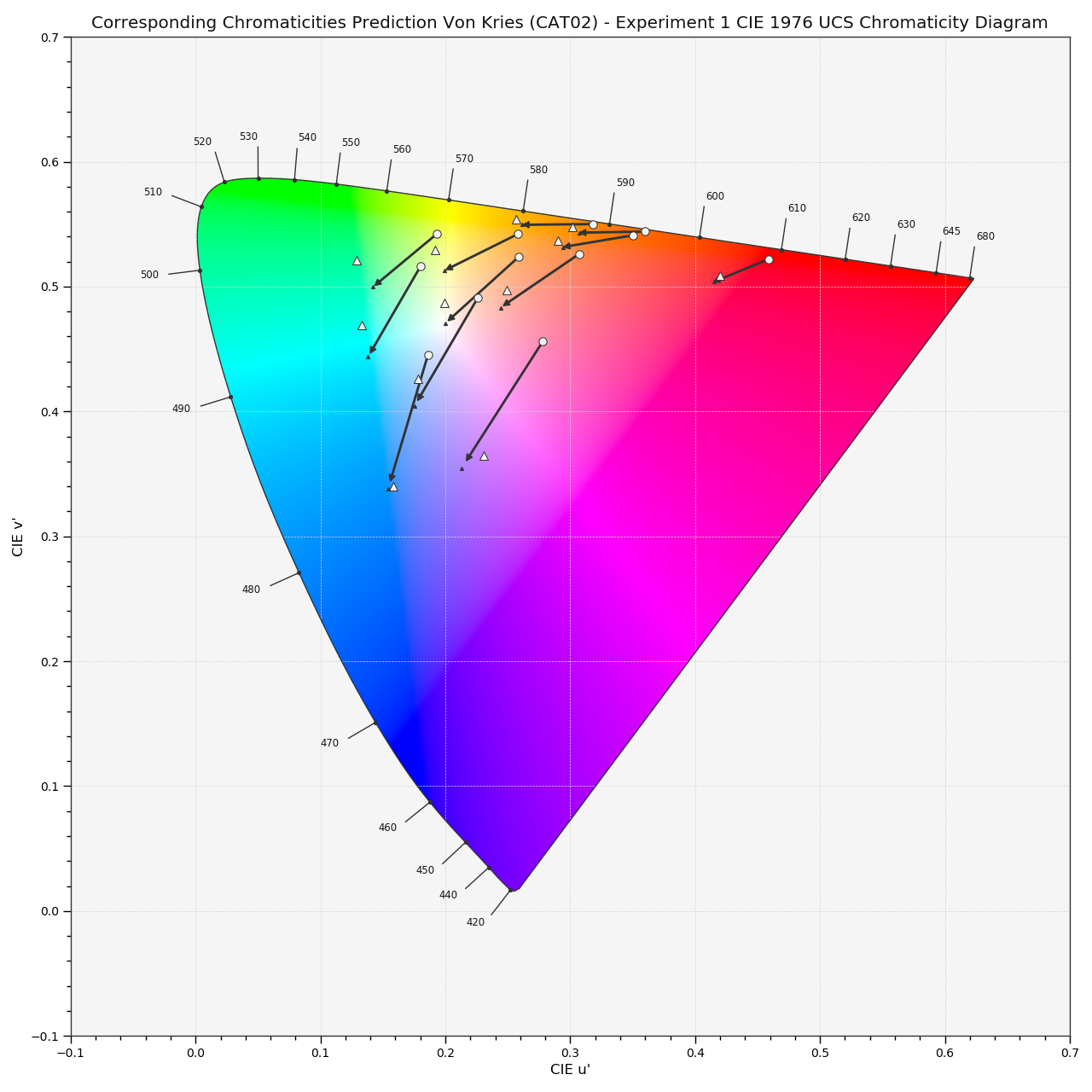# colour.plotting.plot_corresponding_chromaticities_prediction¶

colour.plotting.plot_corresponding_chromaticities_prediction(experiment=1, model='Von Kries', transform='CAT02', **kwargs)[source]

Plots given chromatic adaptation model corresponding chromaticities prediction.

Parameters: Other Parameters: experiment (int, optional) – Corresponding chromaticities prediction experiment number. model (unicode, optional) – Corresponding chromaticities prediction model name. transform (unicode, optional) – Transformation to use with Von Kries chromatic adaptation model. **kwargs (dict, optional) – {colour.plotting.artist(), colour.plotting.diagrams.plot_chromaticity_diagram(), colour.plotting.render()}, Please refer to the documentation of the previously listed definitions. Current figure and axes. tuple

Examples

>>> plot_corresponding_chromaticities_prediction(1, 'Von Kries', 'CAT02')
... # doctest: +SKIP